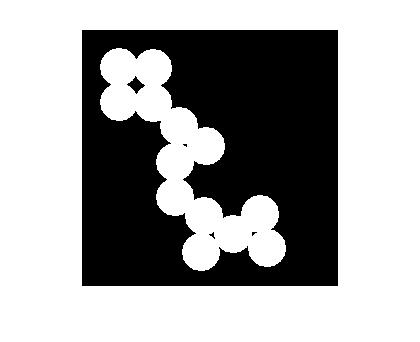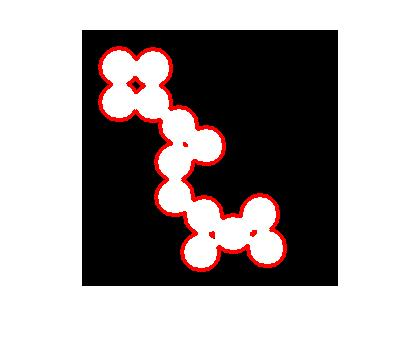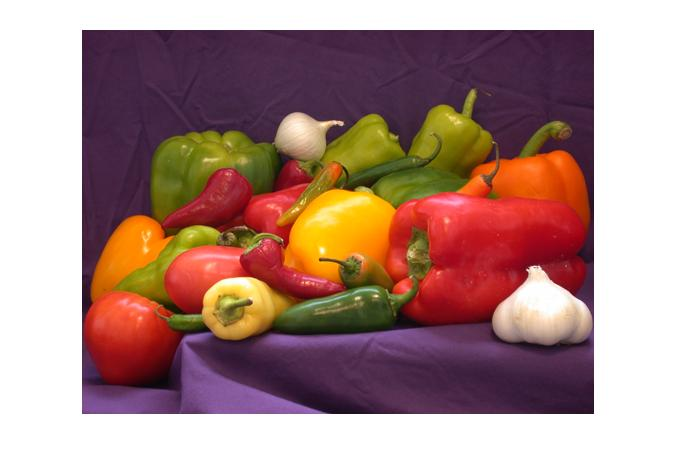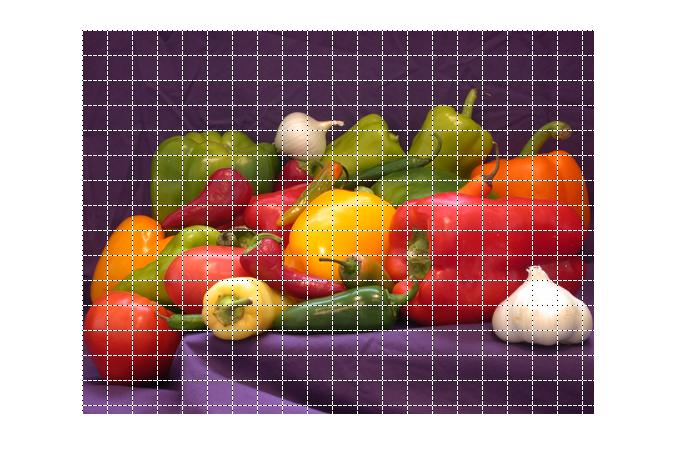# Superimposing line plots on images

Several people have asked me recently how to plot some kind of shape on top of an image, so I thought I'd show the basic technique here. Essentially, you display the image, then call hold on, and then use plot or some other graphics command to superimpose the desired shape. For my first example, I'll superimpose the boundaries found by the bwboundaries function on top of the original binary image.
bw = imread('circles.png');
b = bwboundaries(bw);
imshow(bw)Now call hold on. This causes subsequent plotting commands to add to what's already in the figure, instead of replacing it.
hold onFinally, call plot to superimpose the boundary locations.
for k = 1:numel(b)
plot(b{k}(:,2), b{k}(:,1), 'r', 'Linewidth', 3)
endIt's good to get in the habit of calling hold off when you're done adding plot elements. That way, the next high-level plotting command will start over, which is usually what is expected. My second example shows how to superimpose a 25-pixel-by-25-pixel grid on an image. (See Natasha's blog comment.) Display the image first, and then call hold on.
clf
rgb = imread('peppers.png');
imshow(rgb)
hold onNow superimpose the grid. To make sure the grid is visible over all pixel colors, I'll use the trick of superimposing two line objects with contrasting colors and line styles. The Pixel Region Tool in the Image Processing Toolbox uses this same trick.
M = size(rgb,1);
N = size(rgb,2);

for k = 1:25:M
x = [1 N];
y = [k k];
plot(x,y,'Color','w','LineStyle','-');
plot(x,y,'Color','k','LineStyle',':');
end

for k = 1:25:N
x = [k k];
y = [1 M];
plot(x,y,'Color','w','LineStyle','-');
plot(x,y,'Color','k','LineStyle',':');
end

hold offPublished with MATLAB® 7.3
|

### Comments

To leave a comment, please click here to sign in to your MathWorks Account or create a new one.